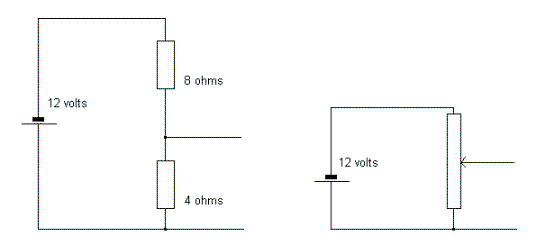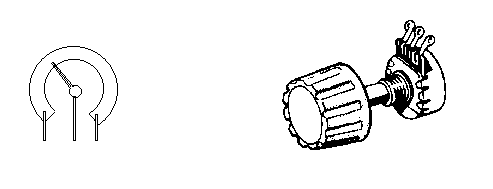Home > Electronics Tutorials > Direct Current (DC) Theory > Potential Dividers Tutorial

Direct Current (DC) Theory - Electronics Teaching

# Potential Dividers Tutorial

Make sure that you understand Ohms Law before reading this.The total resistance of the two series resistors is 8 ohms + 4 ohms = 12 ohms.

The current flowing around the circuit is 12 volts/12 ohms = 1 amp (Ohms Law).

The voltage across the 8 ohm is 1 amp x 8 ohms = 8 volts (again Ohms Law).

The voltage across the 4 ohm is 1 amp x 4 ohms = 4 volts (Mr Ohm again).

The 12 volts of the battery has been divided into 8 volts and 4 volts.

By selecting values for the two resistors, the 12 volts can be divided into any two voltages which add up to 12 volts.

For example, 3 volts and 9 volts, 6 volts and 6 volts etc.

A circuit requiring less than 12 volts can be connected across the lower resistor, as long as it requires a current much lower than the current through the two resistors. If a cardboard strip, coated with carbon, is connected across the battery, together with a "wiper" which can be moved up or down the strip, then you have a POTENTIOMETER (POT for short.) With the wiper at the top then the output is 12 volts. With the wiper at the bottom then the output is zero volts. Any output between 12 volts and zero can be obtained by positioning the wiper correctly.In practice, the strip is curved and the wiper is joined to a spindle which rotates the wiper on the strip when a control knob is twisted. In the drawings, the wiper is the centre connection. Most front panel controls such volume and brightness are "pots". Their purpose is to adjust the voltage fed from one stage to the next.